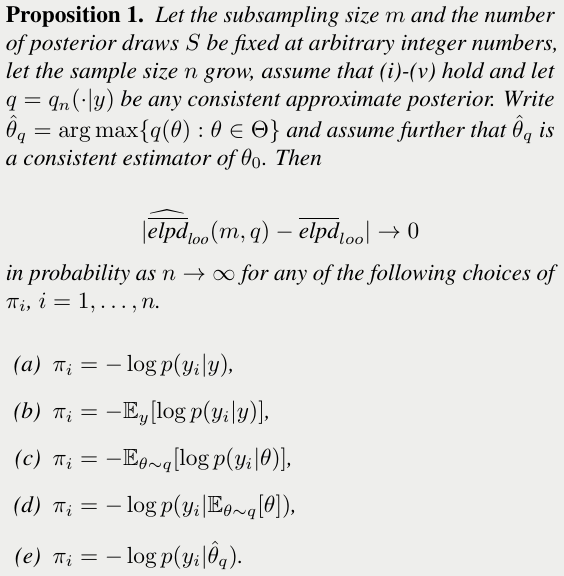# Bayesian Leave-One-Out Cross Validation

##### Posted on Oct 20, 20210 Comments

LOO-CV does not scale well to large datasets.

propose a combination of using approximate inference techniques and probability-proportional-to-size-sampling (PPS) for fast LOO-CV model evaluation for large data.

From a Bayesian decision-theoretic point of view, one wants to make a choice $a\in \cA$ (a model $p_M$ when considering model selection), that maximize the expected utility for a utility function $u(a,\cdot)$ as

$\bar u(a) = \int u(a, \tilde y_i) p_t(\tilde y_i)d\tilde y_i\,,$

where $p_t(\tilde y_i)$ is the true prob. dist. generating observation $\tilde y_i$.

The log score function give rise to using the expected log predictive density (elpd) for model inference, defined as

$\overline{elpd}_M = \int \log p_M(\tilde y_i\mid y)p_t(\tilde y_i)d\tilde y_i\,,$

where $\log p_M(\tilde y_i\mid y)$ is the log predictive density for a new observation for the model $M$.

With LOO-CV,

\begin{align*} \overline{elpd}_{loo} &= \frac 1n \sum_{i=1}^n \log p_M(y_i\mid y_{-i})\\ &= \frac 1n \sum_{i=1}^n \log \int p_M(y_i\mid \theta) p_M(\theta\mid y_{-i}) d\theta\\ &= \frac 1n elpd_{loo} \end{align*}

Theoretical properties:

• LOO-CV, just as WAIC, consistent estimate of the true $elpd_M$ for regular and singular models
• LOO-CV is more robust that WAIC in the finite data domain

Two problems:

• many posterior approximation techniques, such as MCMC, does not scale well for large $n$
• computing $elpd_{loo}$ still needs to be computed over $n$ observations

### Pareto-Smoothed Importance Sampling

Estimate $p_M(y_i\mid y_{-i})$ using importance sampling approximation,

$\log \hat p(y_i\mid y_{i-1}) = \log \left( \frac{\frac 1S\sum_{s=1}^Sp_M(y_i\mid \theta_s)r(\theta_s)}{\frac 1S\sum_{s=1}^Sr(\theta_s)} \right)$

where $\theta_s$ draws from the full posterior $p(\theta\mid y)$ and

$r(\theta_s) = \frac{p_M(\theta_s\mid y_{-i})}{p_M(\theta_s\mid y)} \propto \frac{1}{p_M(y_i\mid \theta_s)}\,.$

In this way, only the full posterior is needed.

The ratios $r(\theta_s)$ can be unstable due to a long right tail, then solve it using Pareto-smoothed importance sampling (PSIS).

PSIS-LOO has the same scaling problem as LOO-CV since it requires

• samples from the true posterior (e.g. using MCMC)
• the estimation of the $\elpd_\loo$ contributions from all observations.

### Contributions

• include a correction term to the importance sampling weights
• propose sampling individual $\elpd_\loo$ components with probability-proportional-to-size sampling (PPS) to estimate $\elpd_\loo$.
• asymptotic properties as $n\rightarrow \infty$

## Bayesian LOO-CV

### Estimate $\elpd_\loo$ using Posterior Approximations

Laplace and variational posterior approximations are attractive for fast model comparisons due to their computational scalability.

Laplace approximation approximates the posterior distribution with a multivariate normal distribution $q_\Lap(\theta\mid y)$ with the mean being the mode of the posterior and the covariance the inverse Hessian at the mode.

In variational inference, find the approximation $q$ from family $\cQ$ that is closest to the true posterior in a KL divergence sense. - mean field (MF) variational approximation $q_{MF}(\theta\mid y)$ - full rank (FR) variational approximation $q_{FR}(\theta\mid y)$

Change $r(\theta)$ to

$r(\theta_s) = \frac{p_M(\theta_s\mid y_{-i})}{q_M(\theta_s\mid y)} = \frac{p_M(\theta_s\mid y_{-i})}{p_M(\theta_s\mid y)}\times \frac{p_M(\theta_s\mid y)}{q_M(\theta_s\mid y)}\propto \frac{1}{p_M(y_i\mid \theta_s)} \frac{p_M(\theta_s\mid y)}{q_M(\theta_s\mid y)}\,.$

### Probability-Proportional-to-Size Subsampling and Hansen-Hurwitz Estimation

using a sample of the $\elpd_\loo$ components to estimate $\elpd_\loo$

sample $m < n$ observations proportional to $\tilde \pi_i\propto \pi_i = -\log p_M(y_i\mid y) = -\log\int p_M(y_i\mid \theta)p_M(\theta\mid y)d\theta$.

In the case of regular models and large $n$, we can approximate $\log p_M(y_i\mid y)\approx \log p_M(y_i\mid \hat\theta)$ where $\hat\theta$ can be a Laplace posterior mean estimate $\hat\theta_q$ or a VI mean estimate $E_{\theta\sim q}[\theta]$.

The estimator for $\elpd_\loo$ can be formulated as

$\widehat{\overline{\elpd}_{\loo, q}} = \frac 1n \frac 1m \sum_{i}^m \frac{1}{\tilde \pi_i}\log \hat p(y_i\mid y_{-i})\,.$Published in categories Note